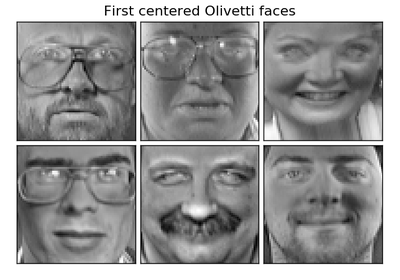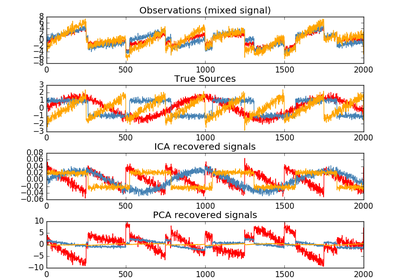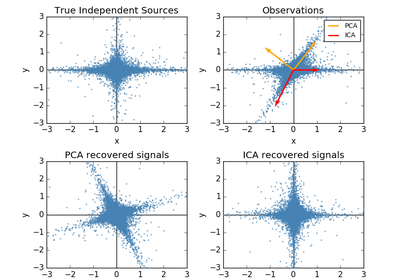# `sklearn.decomposition`.FastICA¶

class `sklearn.decomposition.``FastICA`(n_components=None, algorithm='parallel', whiten=True, fun='logcosh', fun_args=None, max_iter=200, tol=0.0001, w_init=None, random_state=None)[source]

FastICA: a fast algorithm for Independent Component Analysis.

Read more in the User Guide.

Parameters: n_components : int, optional Number of components to use. If none is passed, all are used. algorithm : {‘parallel’, ‘deflation’} Apply parallel or deflational algorithm for FastICA. whiten : boolean, optional If whiten is false, the data is already considered to be whitened, and no whitening is performed. fun : string or function, optional. Default: ‘logcosh’ The functional form of the G function used in the approximation to neg-entropy. Could be either ‘logcosh’, ‘exp’, or ‘cube’. You can also provide your own function. It should return a tuple containing the value of the function, and of its derivative, in the point. Example: def my_g(x): return x ** 3, 3 * x ** 2 fun_args : dictionary, optional Arguments to send to the functional form. If empty and if fun=’logcosh’, fun_args will take value {‘alpha’ : 1.0}. max_iter : int, optional Maximum number of iterations during fit. tol : float, optional Tolerance on update at each iteration. w_init : None of an (n_components, n_components) ndarray The mixing matrix to be used to initialize the algorithm. random_state : int or RandomState Pseudo number generator state used for random sampling. components_ : 2D array, shape (n_components, n_features) The unmixing matrix. mixing_ : array, shape (n_features, n_components) The mixing matrix. n_iter_ : int If the algorithm is “deflation”, n_iter is the maximum number of iterations run across all components. Else they are just the number of iterations taken to converge.

Notes

Implementation based on A. Hyvarinen and E. Oja, Independent Component Analysis: Algorithms and Applications, Neural Networks, 13(4-5), 2000, pp. 411-430

Methods

 `fit`(X[, y]) Fit the model to X. `fit_transform`(X[, y]) Fit the model and recover the sources from X. `get_params`([deep]) Get parameters for this estimator. `inverse_transform`(X[, copy]) Transform the sources back to the mixed data (apply mixing matrix). `set_params`(\*\*params) Set the parameters of this estimator. `transform`(X[, y, copy]) Recover the sources from X (apply the unmixing matrix).
`__init__`(n_components=None, algorithm='parallel', whiten=True, fun='logcosh', fun_args=None, max_iter=200, tol=0.0001, w_init=None, random_state=None)[source]
`fit`(X, y=None)[source]

Fit the model to X.

Parameters: X : array-like, shape (n_samples, n_features) Training data, where n_samples is the number of samples and n_features is the number of features. self :
`fit_transform`(X, y=None)[source]

Fit the model and recover the sources from X.

Parameters: X : array-like, shape (n_samples, n_features) Training data, where n_samples is the number of samples and n_features is the number of features. X_new : array-like, shape (n_samples, n_components)
`get_params`(deep=True)[source]

Get parameters for this estimator.

Parameters: deep : boolean, optional If True, will return the parameters for this estimator and contained subobjects that are estimators. params : mapping of string to any Parameter names mapped to their values.
`inverse_transform`(X, copy=True)[source]

Transform the sources back to the mixed data (apply mixing matrix).

Parameters: X : array-like, shape (n_samples, n_components) Sources, where n_samples is the number of samples and n_components is the number of components. copy : bool (optional) If False, data passed to fit are overwritten. Defaults to True. X_new : array-like, shape (n_samples, n_features)
`set_params`(**params)[source]

Set the parameters of this estimator.

The method works on simple estimators as well as on nested objects (such as pipelines). The latter have parameters of the form `<component>__<parameter>` so that it’s possible to update each component of a nested object.

Returns: self :
`transform`(X, y=None, copy=True)[source]

Recover the sources from X (apply the unmixing matrix).

Parameters: X : array-like, shape (n_samples, n_features) Data to transform, where n_samples is the number of samples and n_features is the number of features. copy : bool (optional) If False, data passed to fit are overwritten. Defaults to True. X_new : array-like, shape (n_samples, n_components)

## Examples using `sklearn.decomposition.FastICA`¶Faces dataset decompositionsBlind source separation using FastICAFastICA on 2D point clouds